International
Tables for
Crystallography
Volume B
Reciprocal space
Edited by U. Shmueli

International Tables for Crystallography (2006). Vol. B. ch. 2.4, pp. 271-272   | 1 | 2 |

## Section 2.4.4.4. Treatment of errors in phase evaluation: Blow and Crick formulation

M. Vijayana* and S. Ramaseshanb

aMolecular Biophysics Unit, Indian Institute of Science, Bangalore 560 012, India, and bRaman Research Institute, Bangalore 560 080, India
Correspondence e-mail:  mv@mbu.iisc.ernet.in

#### 2.4.4.4. Treatment of errors in phase evaluation: Blow and Crick formulation

| top | pdf |

As shown in Section 2.4.2.3, ideally, protein phase angles can be evaluated if two isomorphous heavy-atom derivatives are available. However, in practice, conditions are far from ideal on account of several factors such as imperfect isomorphism, errors in the estimation of heavy-atom parameters, and the experimental errors in the measurement of intensity from the native and the derivative crystals. It is therefore desirable to use as many derivatives as are available for phase determination. When isomorphism is imperfect and errors exist in data and heavy-atom parameters, all the circles in a Harker diagram would not intersect at a single point; instead, there would be a distribution of intersections, such as that illustrated in Fig. 2.4.4.1. Consequently, a unique solution for the phase angle cannot be deduced.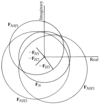Figure 2.4.4.1 | top | pdf |Distribution of intersections in the Harker construction under non-ideal conditions.

The statistical procedure for computing protein phase angles using multiple isomorphous replacement (MIR) was derived by Blow & Crick (1959). In their treatment, Blow and Crick assume, for mathematical convenience, that all errors, including those arising from imperfect isomorphism, could be considered as residing in the magnitudes of the derivative structure factors only. They further assume that these errors could be described by a Gaussian distribution. With these simplifying assumptions, the statistical procedure for phase determination could be derived in the following manner.

Consider the vector diagram, shown in Fig. 2.4.4.2, for a reflection from the ith derivative for an arbitrary valuefor the protein phase angle. Then,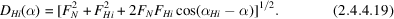Ifcorresponds to the true protein phase angle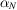, then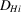coincides with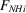. The amount by which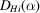differs from, namely,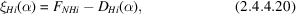is a measure of the departure offrom.is called the lack of closure. The probability forbeing the correct protein phase angle could now be defined as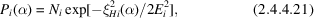whereis the normalization constant and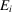is the estimated r.m.s. error. The methods for estimatingwill be outlined later. When several derivatives are used for phase determination, the total probability of the phase anglebeing the protein phase angle would be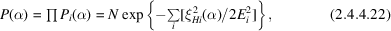where the summation is over all the derivatives. A typical distribution of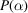plotted around a circle of unit radius is shown in Fig. 2.4.4.3. The phase angle corresponding to the highest value ofwould obviously be the most probable protein phase,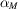, of the given reflection. The most probable electron-density distribution is obtained if eachis associated with the correspondingin a Fourier synthesis.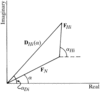Figure 2.4.4.2 | top | pdf |Vector diagram indicating the calculated structure factor,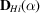, of the ith heavy-atom derivative for an arbitrary valuefor the phase angle of the structure factor of the native protein.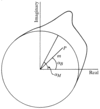Figure 2.4.4.3 | top | pdf |The probability distribution of the protein phase angle. The point P is the centroid of the distribution.

Blow and Crick suggested a different way of using the probability distribution. In Fig. 2.4.4.3, the centroid of the probability distribution is denoted by P. The polar coordinates of P are m and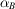, where m, a fractional positive number with a maximum value of unity, andare referred to as the figure of merit' and the best phase', respectively. One can then compute a `best Fourier' with coefficients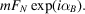The best Fourier is expected to provide an electron-density distribution with the lowest r.m.s. error. The figure of merit and the best phase are usually calculated using the equations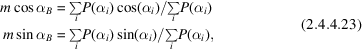where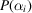are calculated, say, at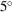intervals (Dickerson et al., 1961). The figure of merit is statistically interpreted as the cosine of the expected error in the calculated phase angle and it is obviously a measure of the precision of phase determination. In general, m is high whenandare close to each other and low when they are far apart.

### ReferencesBlow, D. M. & Crick, F. H. C. (1959). The treatment of errors in the isomorphous replacement method. Acta Cryst. 12, 794–802.Google ScholarDickerson, R. E., Kendrew, J. C. & Strandberg, B. E. (1961). The crystal structure of myoglobin: phase determination to a resolution of 2 Å by the method of isomorphous replacement. Acta Cryst. 14, 1188–1195.Google Scholar